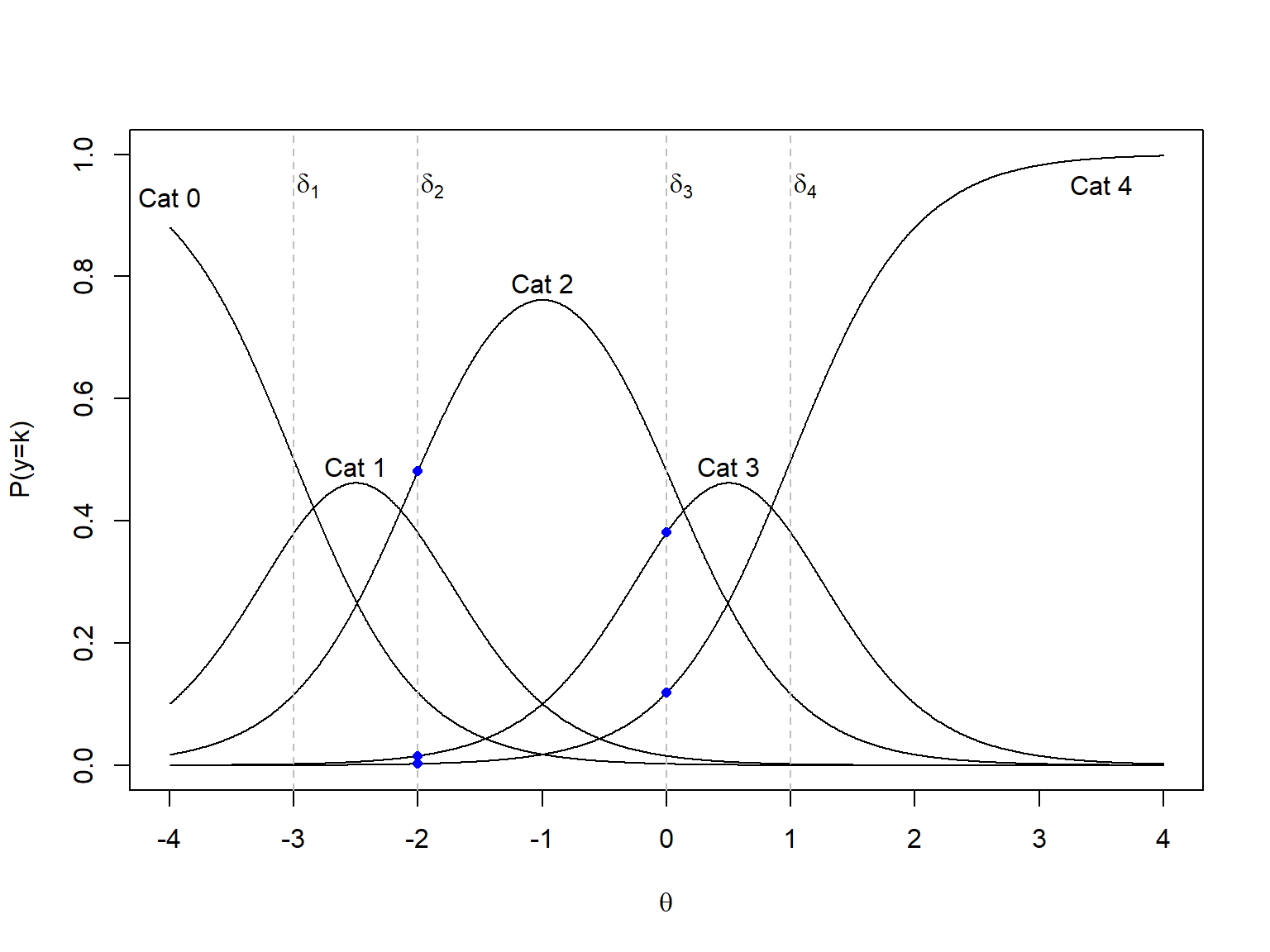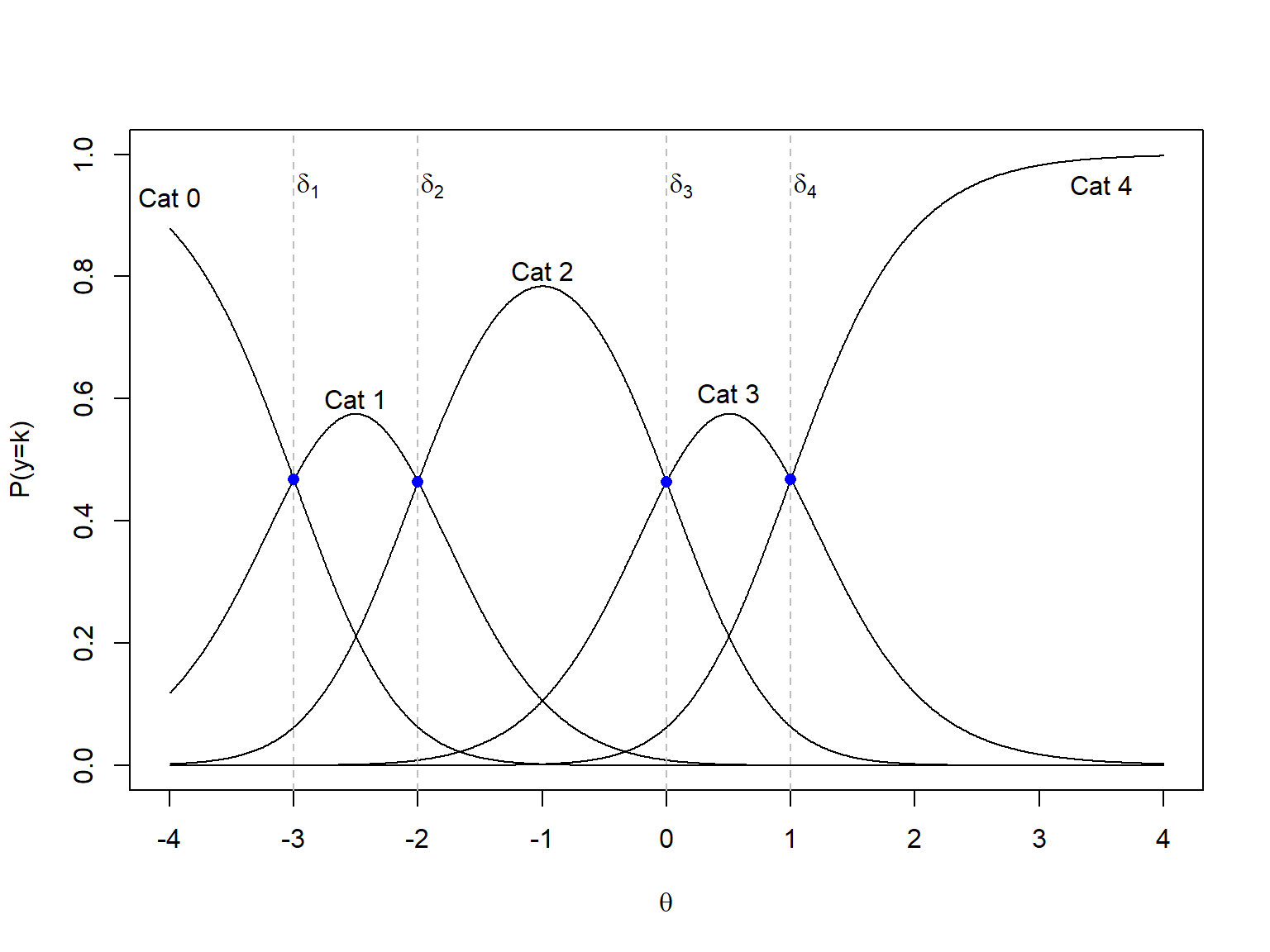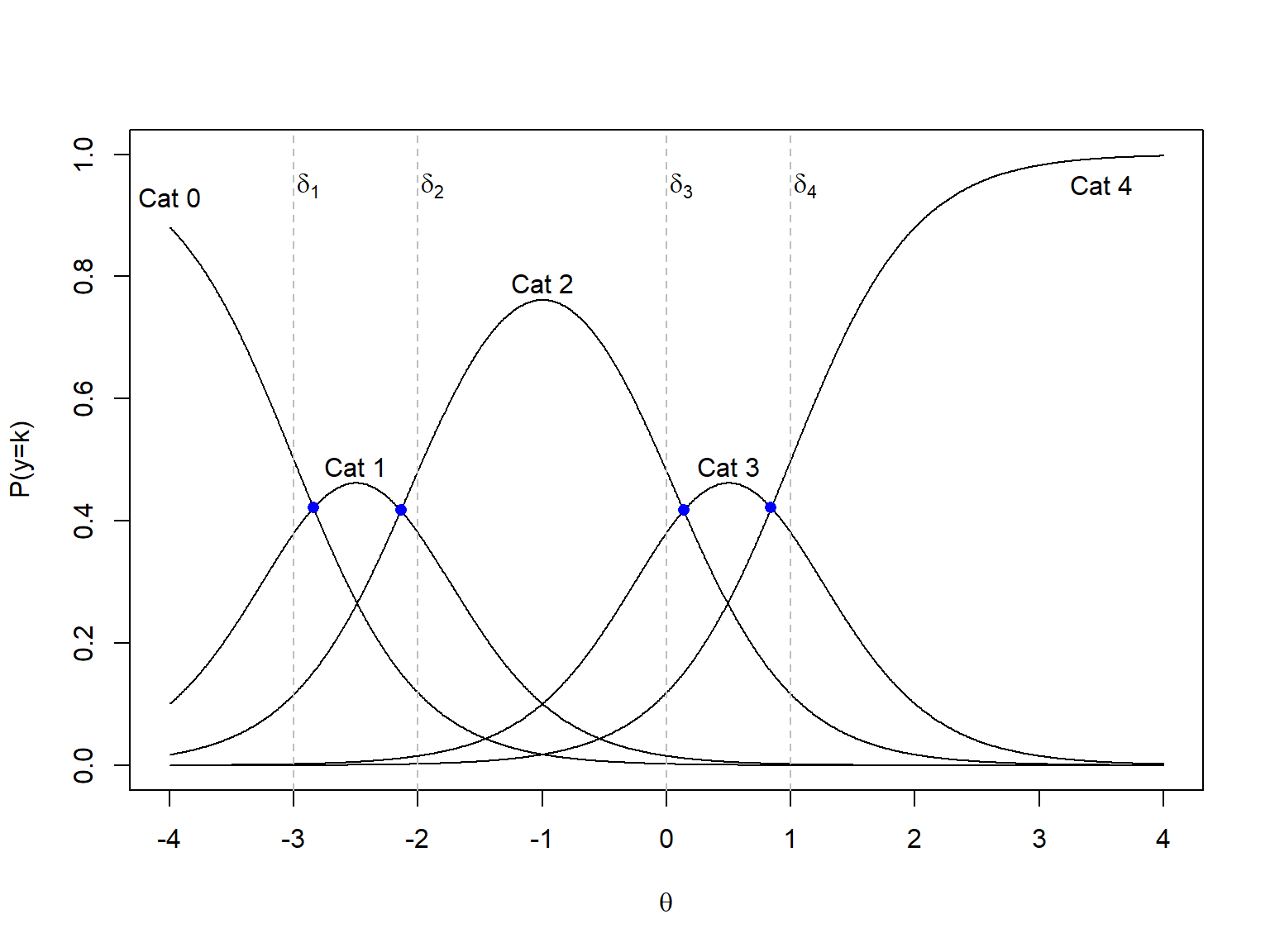# Intersection Points Between Two Adjacent Categories in the Graded Response Model

The interpretation of between-category thresholds in the Graded Response Model is different than the step difficulty parameters in the RSM/PCM/GPCM family due to a different functional form. While the step parameters in the RSM/PCM/GPCM family represent the point on the latent trait continuum where one category becomes more likely than the previous category, it is not the same for between-category thresholds in the Graded Response Model. So, this post is my response to a curious student who wondered at what point on the latent trait continuum the intersections occur between two adjacent categories for the Graded Response Model.

Cengiz Zopluoglu (University of Miami)
2-26-2020

One of the IRT models available for polytomous response outcomes (response outcomes with more than two categories) is the Graded Response Model (GRM). GRM can be used for questionnaires with common rating scale items in which item responses are ordered categorical responses such as Strongly Disagree (0), Disagree (1), Agree (2), and Strongly Agree (3).

In GRM, for an item with $$K$$ categories (k=0,1,2,…K), the probability of selecting a response category j (j=1,2,..K) or above is defined as:

$P(y\geq j)=\frac{1}{1+e^{a(\delta_j-\theta)}},$ where $$a$$ is the item discrimination, $$\delta_j$$ is the between-category threshold between category $$j-1$$ and category $$j$$, and $$\theta$$ is the latent trait for the respondent. The number of between-category thresholds for each item is equal to K-1. The probability of selecting each response category is subsequently defined as:

$P(y=0) = 1 - P(y\geq 1)$ $P(y=1) = P(y\geq 1) - P(y\geq 2)$ $P(y=2) = P(y\geq 2) - P(y\geq 3)$ $...$ $P(y=K)=P(y\geq K).$

Below is a plot called Category Response Curves for a hypothetical 5-point rating scale item with parameters $$a=2$$, $$\delta_1=-3$$, $$\delta_2=-2$$, $$\delta_3=0$$, and $$\delta_4=1$$.Figure 1: Graded Response Model Category Response Curves

Category Response Curves display the probability of selecting each response category as a function of $$\theta$$. Vertical lines in Figure 1 represents the points on the latent trait continuum, where $$\theta=\delta_1$$, $$\theta=\delta_2$$, $$\theta=\delta_3$$, and $$\theta=\delta_4$$. The interpretation of between-category thresholds in GRM is that they represent the point on the latent trait continuum where the probability of responding to a certain category or above is equal to 0.5. For instance, $$\delta_2=-2$$ means that the probability of choosing Category 2 or above is equal to .5 when $$\theta=-2$$. In other words, the three blue dots on the vertical line where $$\theta=\delta_2=-2$$ represent the probability of responding Category 2, Category 3, and Category 4 and the sum of these three probabilities is equal to 0.5. Or, similarly, the two blue dots on the vertical line where $$\theta=\delta_3=0$$ represents the probability of choosing response category 3 and Category 4 and the sum is equal to 0.5.

The interpretation of the category thresholds (also called step difficulty) is different for a different family of models [Rating Scale Model(RSM), Partial Credit Model(PCM), and Generalized Partial Credit Model(GPCM)]. In GPCM, we can directly write the probability of responding to a certain category:

$P(y=j)=\frac{e^{\sum_{h=0}^{j}a(\theta-\delta_j)}}{\sum_{j=0}^{K}e^{\sum_{h=0}^{j}a(\theta-\delta_j)}}$

The between-category thresholds in GPCM are easier to interpret. They are simply the intersection points between any two adjacent categories. For instance, suppose we plot the Category Response Curves for GPCM for the same set of parameters, $$a=2$$, $$\delta_1=-3$$, $$\delta_2=-2$$, $$\delta_3=0$$, and $$\delta_4=1$$. You will see that the intersection points between two adjacent categories (the point where one category becomes more likely than the previous category) occur exactly when $$\theta=\delta_j$$.Figure 2: Generalized Partial Credit Model Category Response Curves

## Where do the intersection points occur for the Graded Response Model?

Let’s go back to Figure 1. Notice that the intersection points in GRM are happening somewhere else, not necessarily when $$\theta=\delta_j$$. As we talk about these things and the differences between GRM and GPCM, a student asked me “OK, so where do they occur, then?” I didn’t know. I said I would think about it and got back to them. I don’t remember I have ever read anything or seen any equation that would provide the intersection points for GRM. Are they important for any practical reason? Probably no! After the class, I have checked a few IRT textbooks to make sure it is not hidden somewhere as appendix or supplemental information. I couldn’t find anything. So, I decided to spend some time on it.Figure 3: Graded Response Model Category Response Curves

Technically, it should be simple. We know that the probability of responding category $$j$$ is equal to the probability of responding category $$j+1$$ at those intersection points. For instance, we can write the following for the first intersection point:

$P(y=0) = P(y=1)$ $1 - P(y\geq 1) = P(y\geq 1) - P(y\geq 2)$ $2 P(y\geq 1) - P(y\geq 2) - 1 = 0$ $\frac{2}{1+e^{a(\delta_1-\theta)}} - \frac{1}{1+e^{a(\delta_2-\theta)}} - 1 = 0$

Similarly, we can write the following for the second intersection point:

$P(y=1) = P(y=2)$ $P(y\geq 1) - P(y\geq 2) = P(y\geq 2) - P(y\geq 3)$ $P(y\geq 1) - 2 P(y\geq 2) - P(y\geq 3) = 0$ $\frac{1}{1+e^{a(\delta_1-\theta)}} - \frac{2}{1+e^{a(\delta_2-\theta)}} - \frac{1}{1+e^{a(\delta_3-\theta)}} = 0$ Let’s do it one more time for the third intersection point because I want to see a pattern emerging before diving into it.

$P(y=2) = P(y=3)$ $P(y\geq 2) - P(y\geq 3) = P(y\geq 3) - P(y\geq 4)$ $P(y\geq 2) - 2 P(y\geq 3) - P(y\geq 4) = 0$ $\frac{1}{1+e^{a(\delta_2-\theta)}} - \frac{2}{1+e^{a(\delta_3-\theta)}} - \frac{1}{1+e^{a(\delta_4-\theta)}} = 0$ Finally, for the last intersection point:

$P(y=3) = P(y=4)$ $P(y\geq 3) - P(y\geq 4) = P(y\geq 4)$ $P(y\geq 3) - 2 P(y\geq 4) = 0$ $\frac{1}{1+e^{a(\delta_3-\theta)}} - \frac{2}{1+e^{a(\delta_4-\theta)}} = 0$

All we need to do is to solve these equations for $$\theta$$, and that solution should give us the point on the theta continuum where one category becomes more likely than the previous one for GRM. Sounds easy, right? Well, it was ugly.

First, it seems that we can not find a general equation that works for all intersection points because the equation for the first intersection and the equation for the last intersection are structurally different. However, there should be a general equation for any intersection between the first and last intersection as they have similar form of equation. After spending a few hours, pulling my hairs and beard several times, I come up with some equations. For the first intersection:

$I_{0,1}=\frac{ln(\frac{e^{a\delta_1+a\delta_2}}{e^{a\delta_2}-2e^{a\delta_1}})}{a}$

For the last intersection:

$I_{K-1,K}=\frac{ln(e^{a\delta_k}-2e^{a\delta_{k-1}})}{a}$ For any other intersection between two adjacent categories (excluding the first and last intersection):

$I_{j,j+1}=\frac{ln( \frac{e^{a\delta_{j}+a\delta_{j+1}}+e^{a\delta_{j+1}+a\delta_{j+2}}-2e^{a\delta_{j}+a\delta_{j+2}}} {e^{a\delta_{j}}+e^{a\delta_{j+2}}-2e^{a\delta_{j+1}}})} {a}$

I checked them numerically, and they seem to be holding together. I tried but couldn’t simplify them further. I wish I could put them in a nice-looking equation. For those who are interested, this file has some algebra starting from the equations above and solving them for $$\theta$$.

## Appendix

R code for Figure 2


a  = 2
d1 = -3
d2 = -2
d3 = 0
d4 = 1

theta <- seq(from=-4,to=4,.01)

exp0 = 1
exp1 = exp(a*(theta-d1))
exp2 = exp(a*(theta-d1)+a*(theta-d2))
exp3 = exp(a*(theta-d1)+a*(theta-d2)+a*(theta-d3))
exp4 = exp(a*(theta-d1)+a*(theta-d2)+a*(theta-d3)+a*(theta-d4))

p0 = exp0/(exp0+exp1+exp2+exp3+exp4)
p1 = exp1/(exp0+exp1+exp2+exp3+exp4)
p2 = exp2/(exp0+exp1+exp2+exp3+exp4)
p3 = exp3/(exp0+exp1+exp2+exp3+exp4)
p4 = exp4/(exp0+exp1+exp2+exp3+exp4)

plot(theta,p0,type="l",ylim=c(0,1),xlab=expression(theta),ylab="P(y=k)",xaxt='n')
axis(side=1,at=seq(-4,4,1))
points(theta,p1,type="l")
points(theta,p2,type="l")
points(theta,p3,type="l")
points(theta,p4,type="l")
text(-4,.93,"Cat 0")
text(-2.5,.6,"Cat 1")
text(-1,.81,"Cat 2")
text(.5,.61,"Cat 3")
text(3.5,.95,"Cat 4")
abline(v=d1,lty=2,col="gray")
abline(v=d2,lty=2,col="gray")
abline(v=d3,lty=2,col="gray")
abline(v=d4,lty=2,col="gray")

points(d1,p1[which(theta==d1)],type="p",pch=16,cex=1,col="blue")
points(d2,p2[which(theta==d2)],type="p",pch=16,cex=1,col="blue")
points(d3,p3[which(theta==d3)],type="p",pch=16,cex=1,col="blue")
points(d4,p4[which(theta==d4)],type="p",pch=16,cex=1,col="blue")

text(d1+.12,.95,expression(delta))
text(d2+.12,.95,expression(delta))
text(d3+.12,.95,expression(delta))
text(d4+.12,.95,expression(delta))

R code for Figure 3


a  = 2
d1 = -3
d2 = -2
d3 = 0
d4 = 1

theta <- seq(from=-4,to=4,.01)

p1orabove = 1/(1+exp(a*(d1-theta)))
p2orabove = 1/(1+exp(a*(d2-theta)))
p3orabove = 1/(1+exp(a*(d3-theta)))
p4orabove = 1/(1+exp(a*(d4-theta)))

p0 = 1-p1orabove
p1 = p1orabove - p2orabove
p2 = p2orabove - p3orabove
p3 = p3orabove - p4orabove
p4 = p4orabove

plot(theta,p0,type="l",ylim=c(0,1),xlab=expression(theta),ylab="P(y=k)",xaxt='n')
axis(side=1,at=seq(-4,4,1))
points(theta,p1,type="l")
points(theta,p2,type="l")
points(theta,p3,type="l")
points(theta,p4,type="l")
text(-4,.93,"Cat 0")
text(-2.5,.49,"Cat 1")
text(-1,.79,"Cat 2")
text(.5,.49,"Cat 3")
text(3.5,.95,"Cat 4")

int1 = log(exp(a*d1+a*d2)/(exp(a*d2)-2*exp(a*d1)))/a
int2 = log((exp(a*d1+a*d2)+exp(a*d2+a*d3)-(2*exp(a*d1+a*d3)))/(exp(a*d1)+exp(a*d3)-(2*exp(a*d2))))/a
int3 = log((exp(a*d2+a*d3)+exp(a*d3+a*d4)-(2*exp(a*d2+a*d4)))/(exp(a*d2)+exp(a*d4)-(2*exp(a*d3))))/a
int4 = log(exp(a*d4)-2*exp(a*d3))/a

p1orabove = 1/(1+exp(a*(d1-int1)))
p0 = 1-p1orabove
points(int1,p0,type="p",pch=16,cex=1,col="blue")

p1orabove = 1/(1+exp(a*(d1-int2)))
p2orabove = 1/(1+exp(a*(d2-int2)))
p1 = p1orabove-p2orabove
points(int2,p1,type="p",pch=16,cex=1,col="blue")

p2orabove = 1/(1+exp(a*(d2-int3)))
p3orabove = 1/(1+exp(a*(d3-int3)))
p2 = p2orabove-p3orabove
points(int3,p2,type="p",pch=16,cex=1,col="blue")

p3orabove = 1/(1+exp(a*(d3-int4)))
p4orabove = 1/(1+exp(a*(d4-int4)))
p3 = p3orabove-p4orabove
points(int4,p3,type="p",pch=16,cex=1,col="blue")

abline(v=d1,lty=2,col="gray")
abline(v=d2,lty=2,col="gray")
abline(v=d3,lty=2,col="gray")
abline(v=d4,lty=2,col="gray")

text(d1+.12,.95,expression(delta))
text(d2+.12,.95,expression(delta))
text(d3+.12,.95,expression(delta))
text(d4+.12,.95,expression(delta))

### Citation

Zopluoglu (2020, Feb. 26). Cengiz Zopluoglu: Intersection Points Between Two Adjacent Categories in the Graded Response Model. Retrieved from https://github.com/czopluoglu/website/tree/master/docs/posts/grmint/
@misc{zopluoglu2020intersection,
}